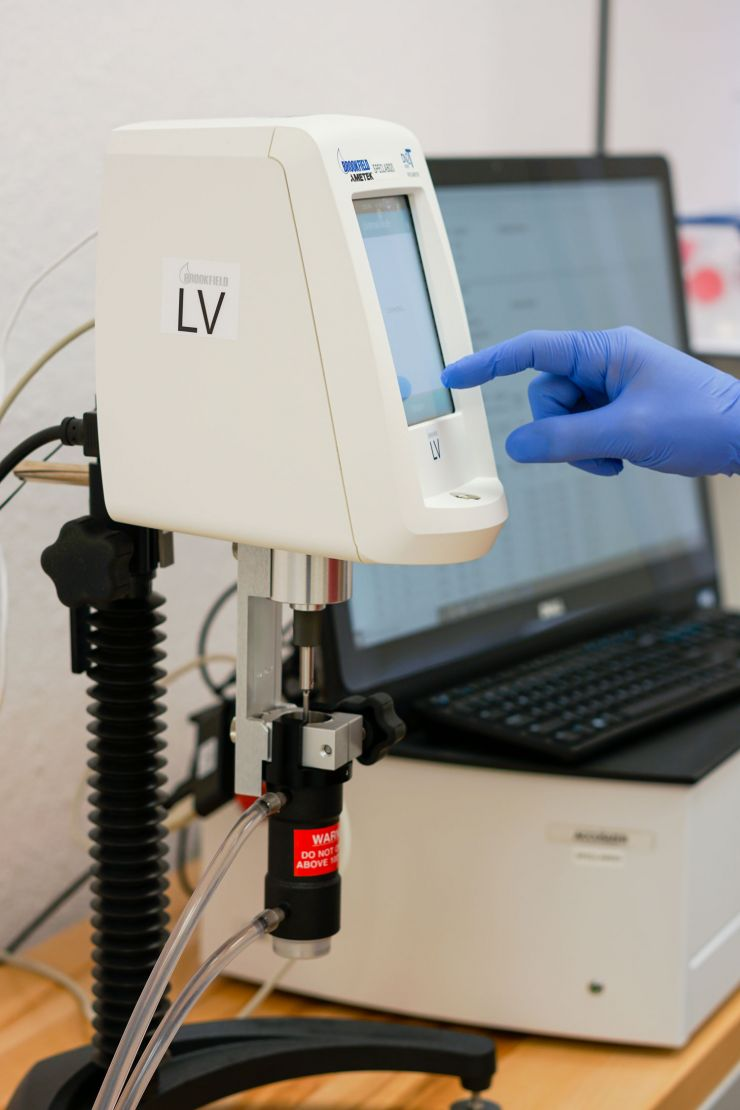# Viscosity#### Viscosity USP <912>

Viscosity is a measure of how “thick” or viscous a liquid or cream is. The viscosity is dependent on the temperature of the liquid being measured (think of how “thick" molasses is when cold or hot). We measure the viscosity by means of rheometry, which is measured by calculating the force acting on a rotor (torque) when rotating at a constant rotational speed in a liquid.

Specifically we test viscosity using Method II. Concentric Cylinder Rheometers. This method determines the viscosity by introducing a fluid into a two cylinder system, a rotating cylinder and a stationary cylinder. The gap between the inner and outer cylinders is filled by the fluid. The viscosity of the fluid is then measured by the shear stress (torque on the rotor axis) resulting from a given shear rate (or rotational speed).
USP <912>

\$ 75